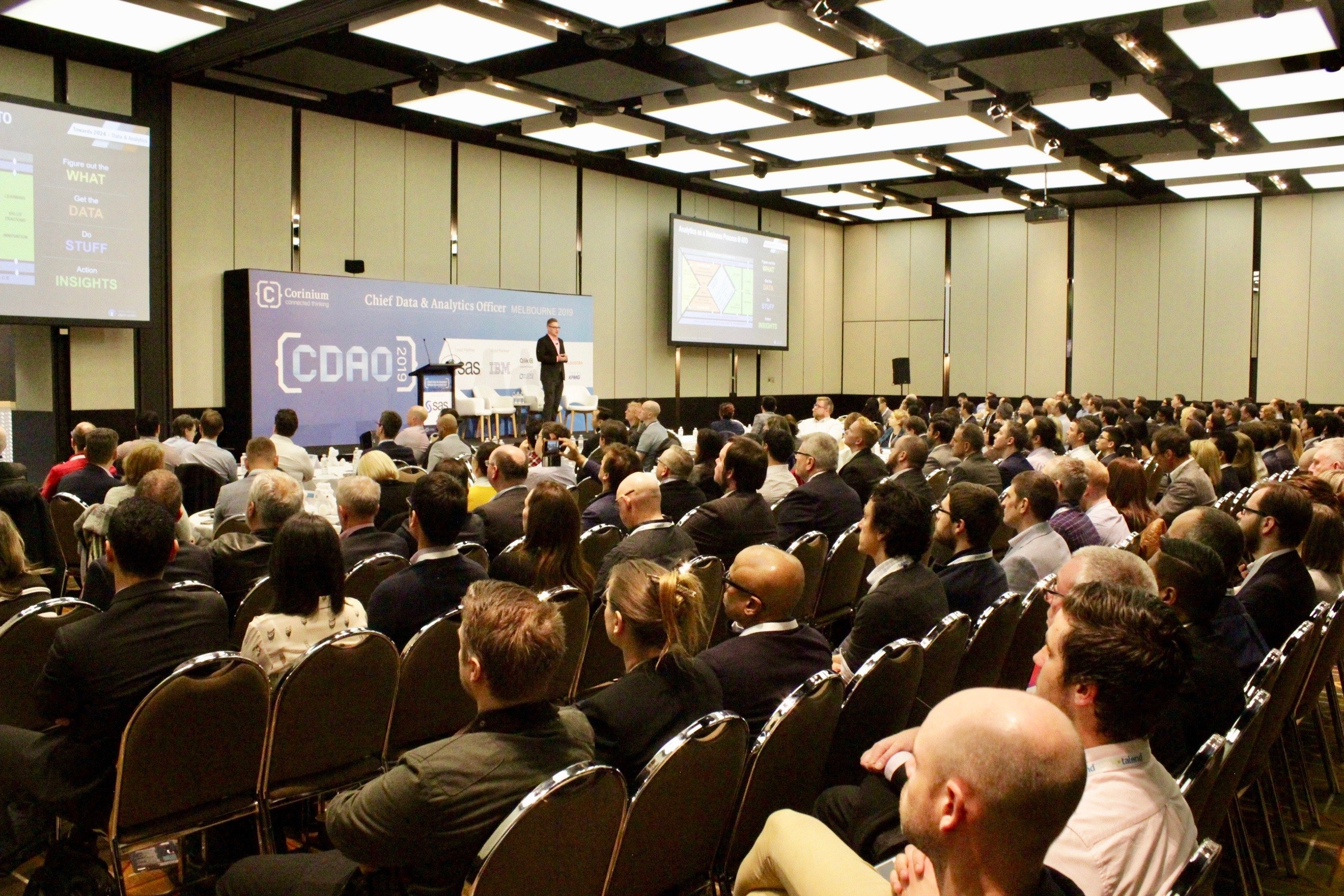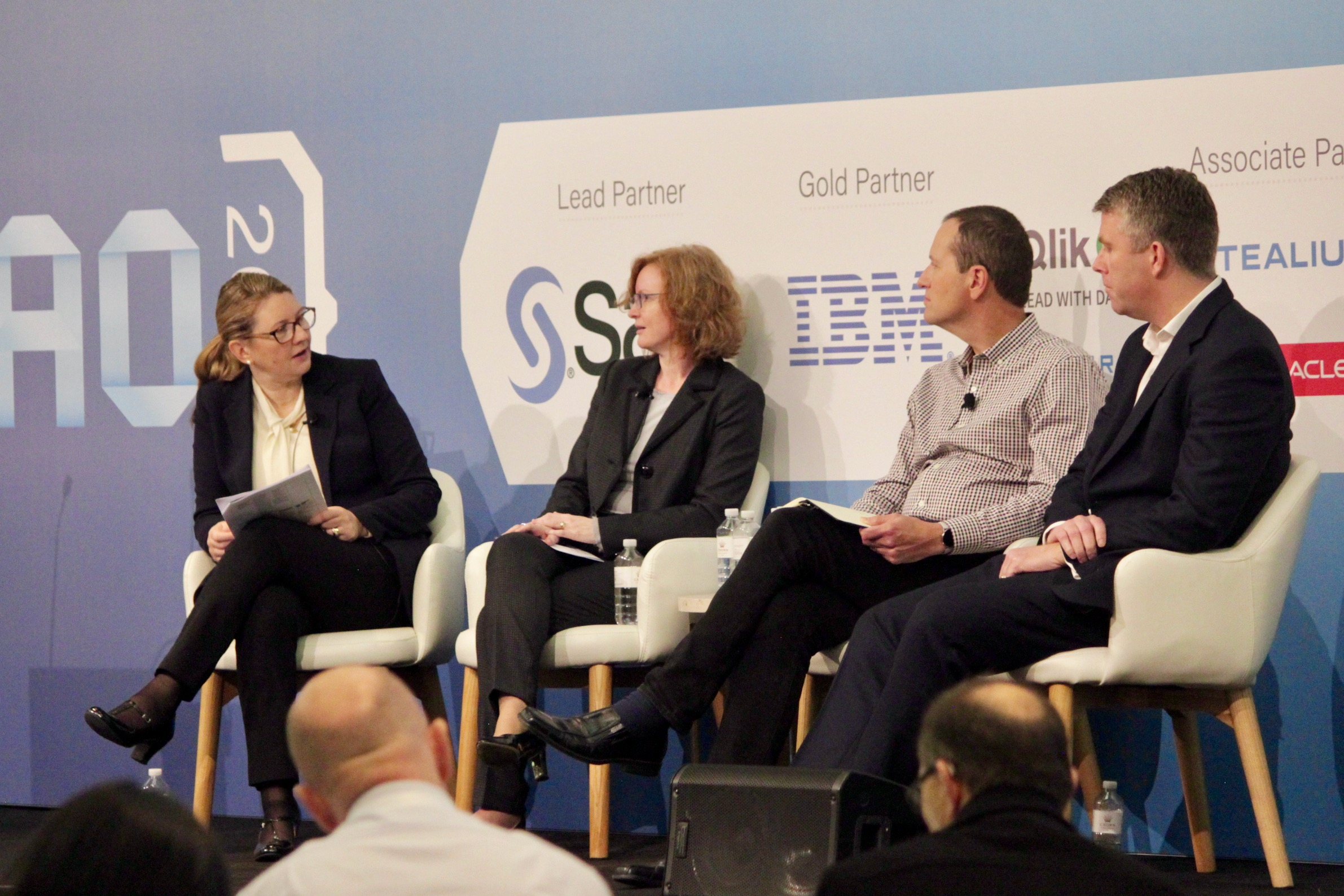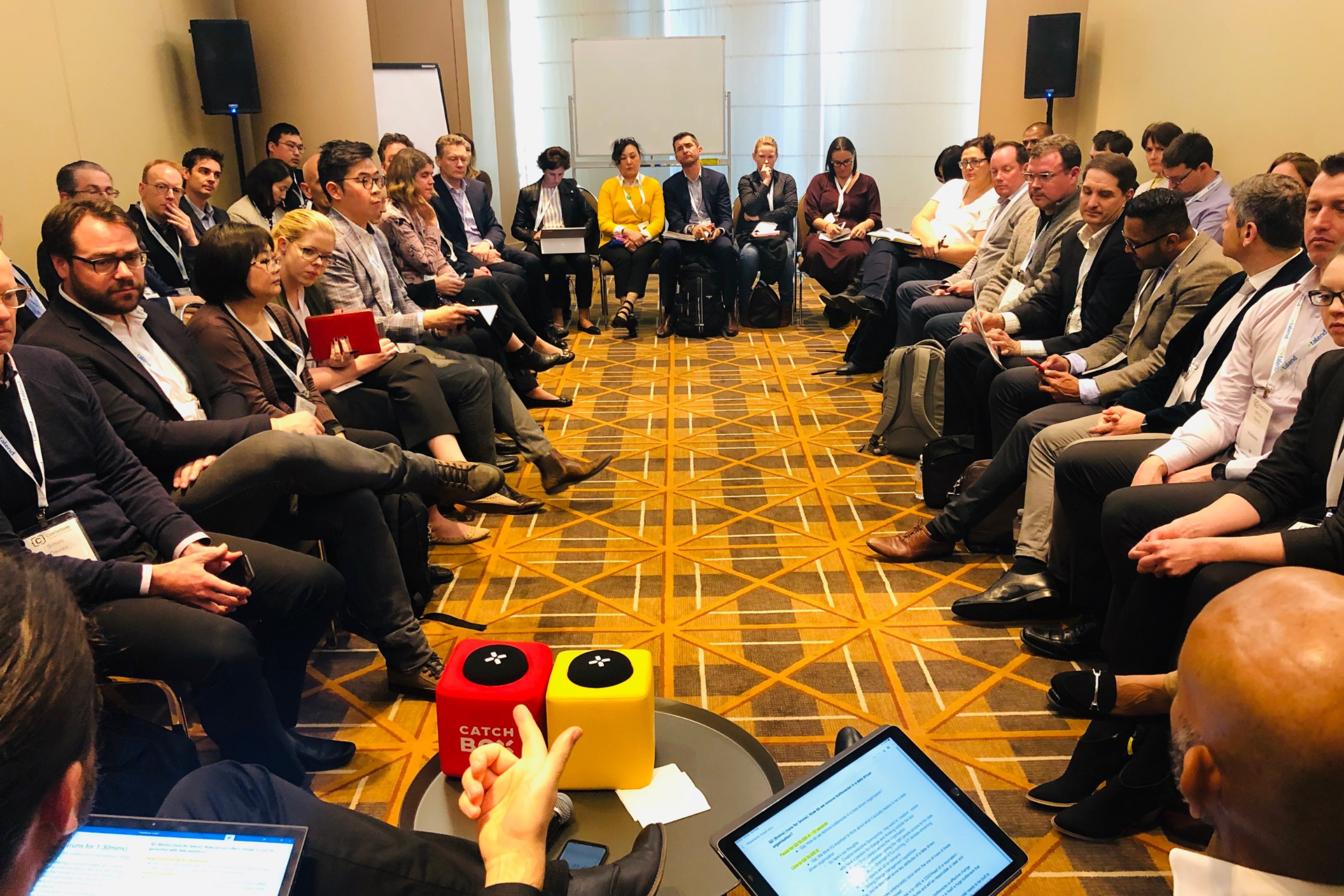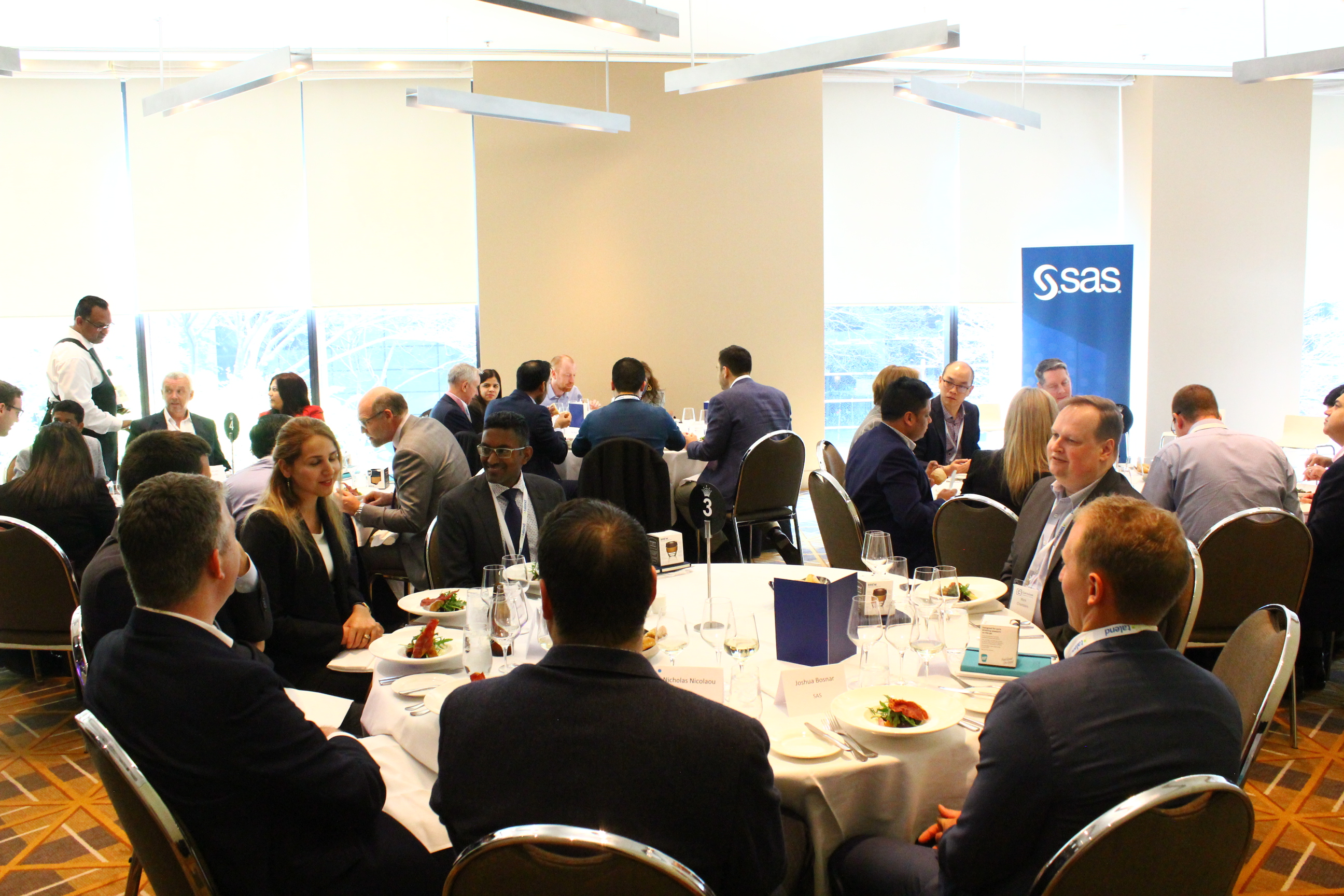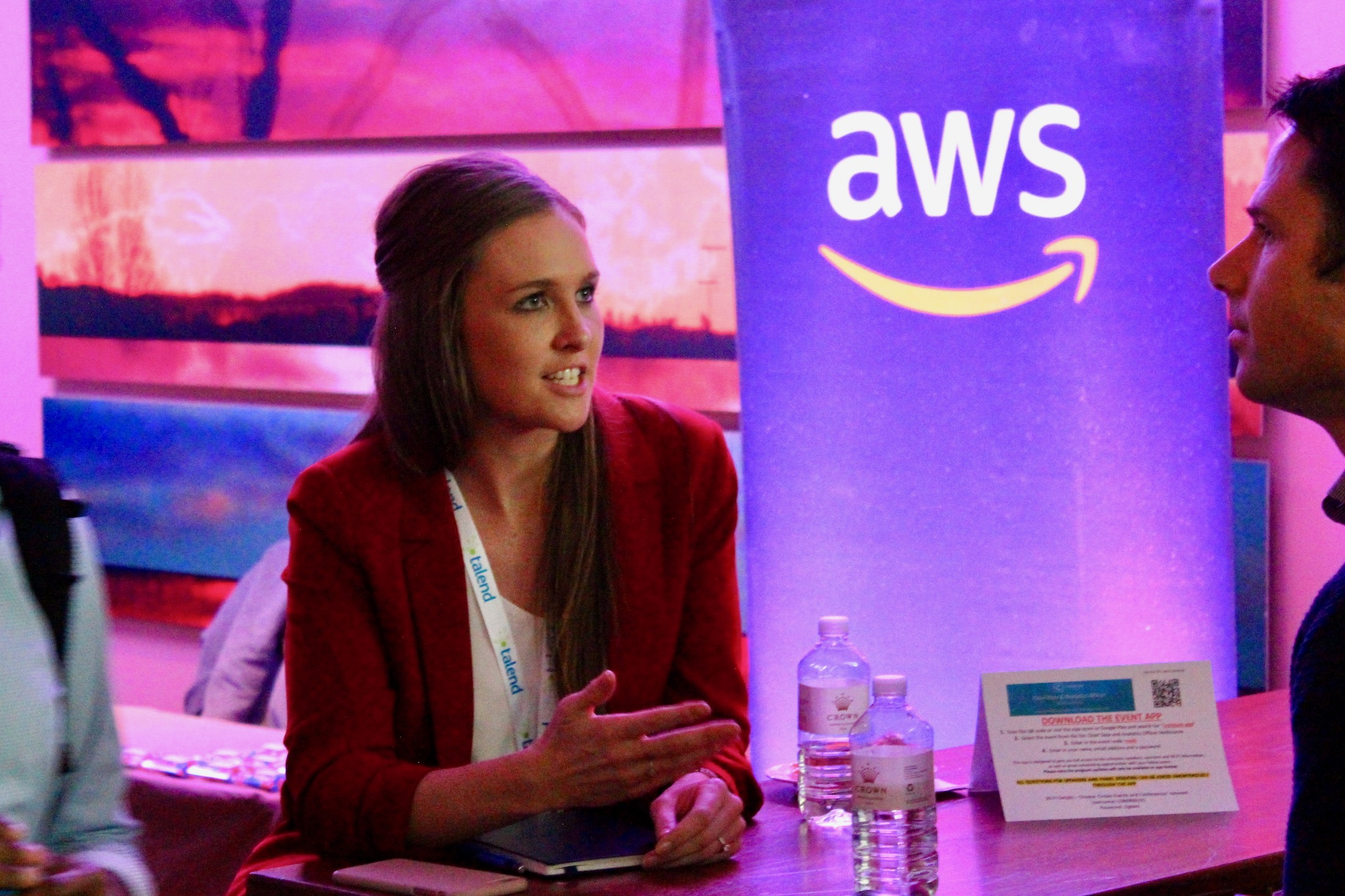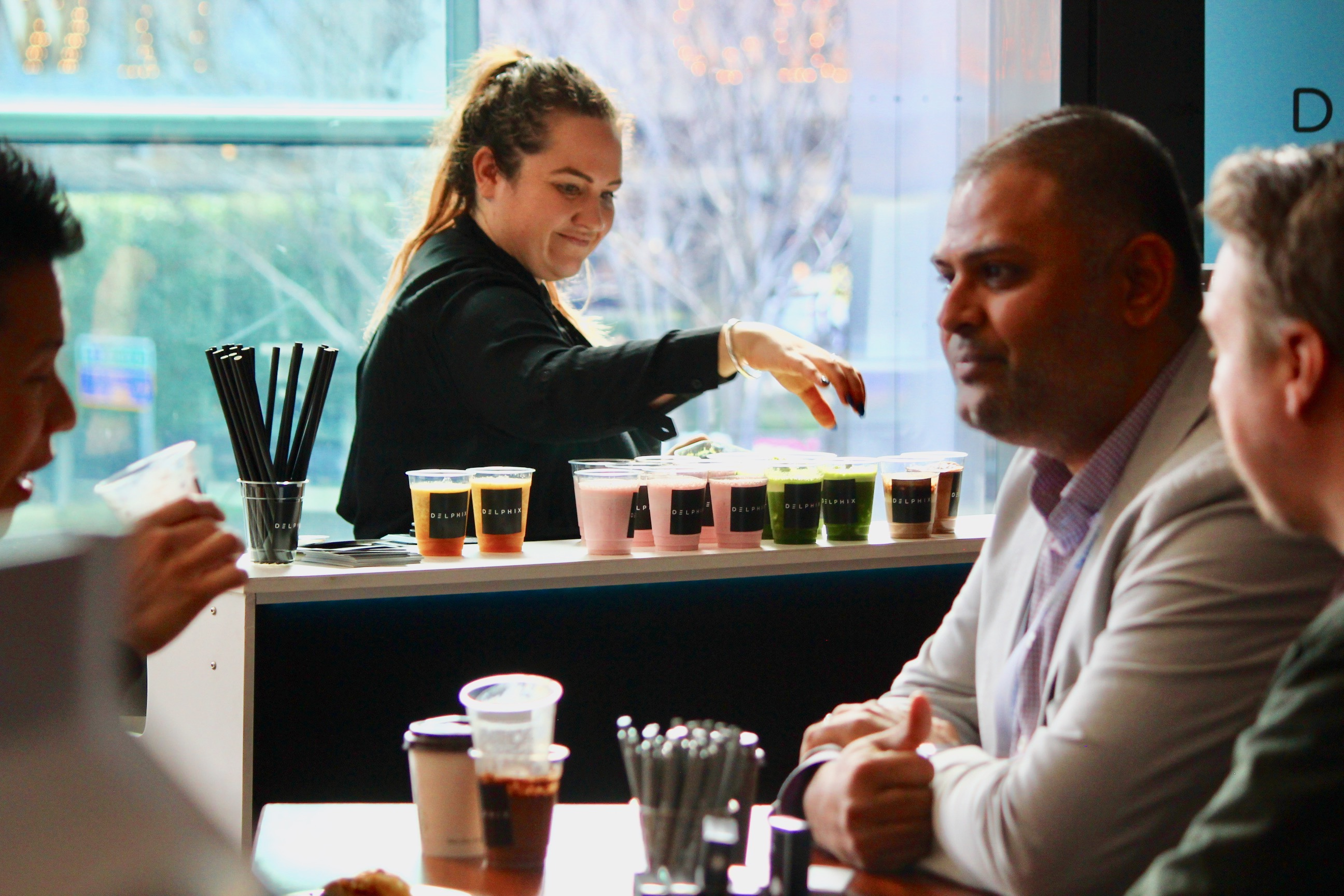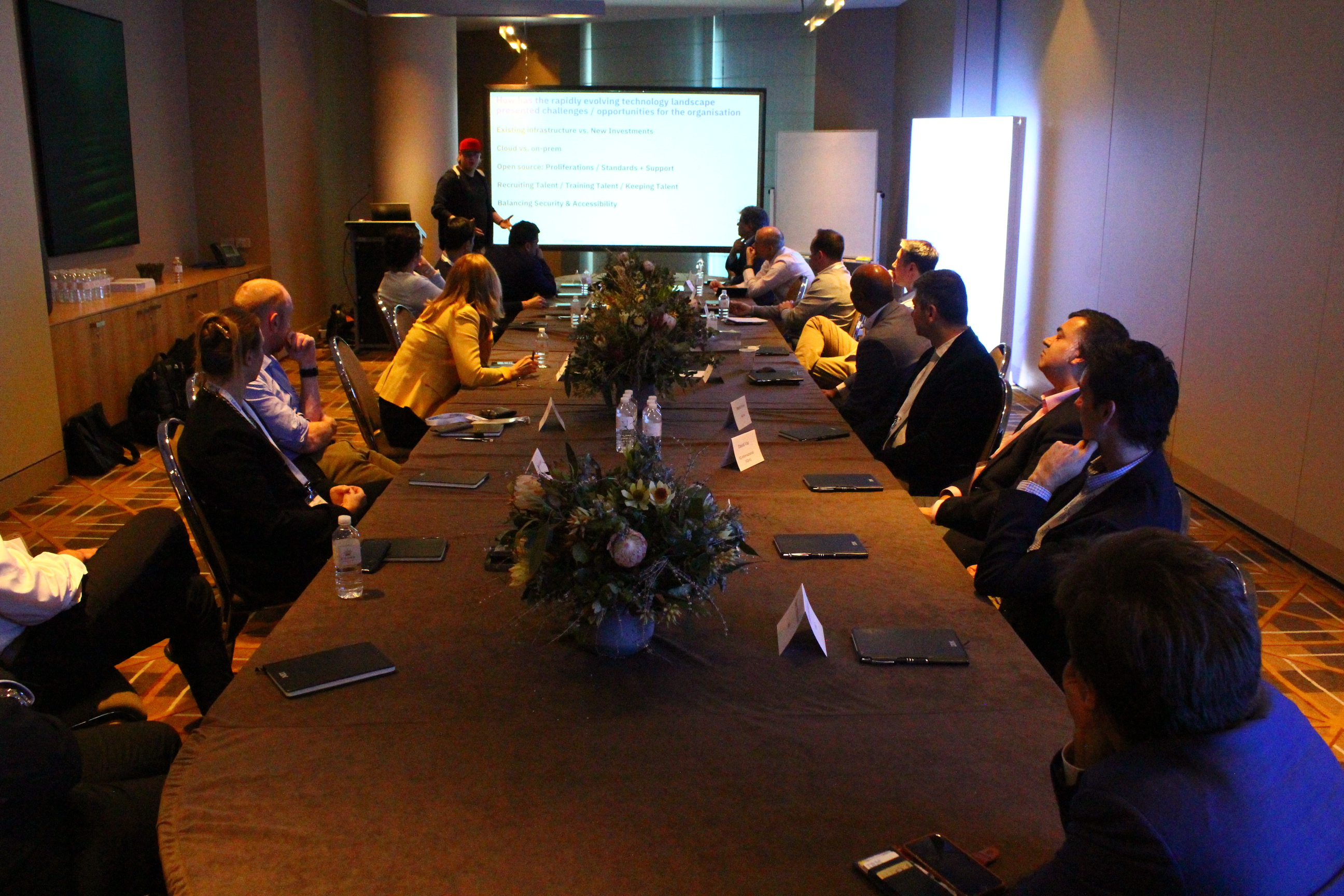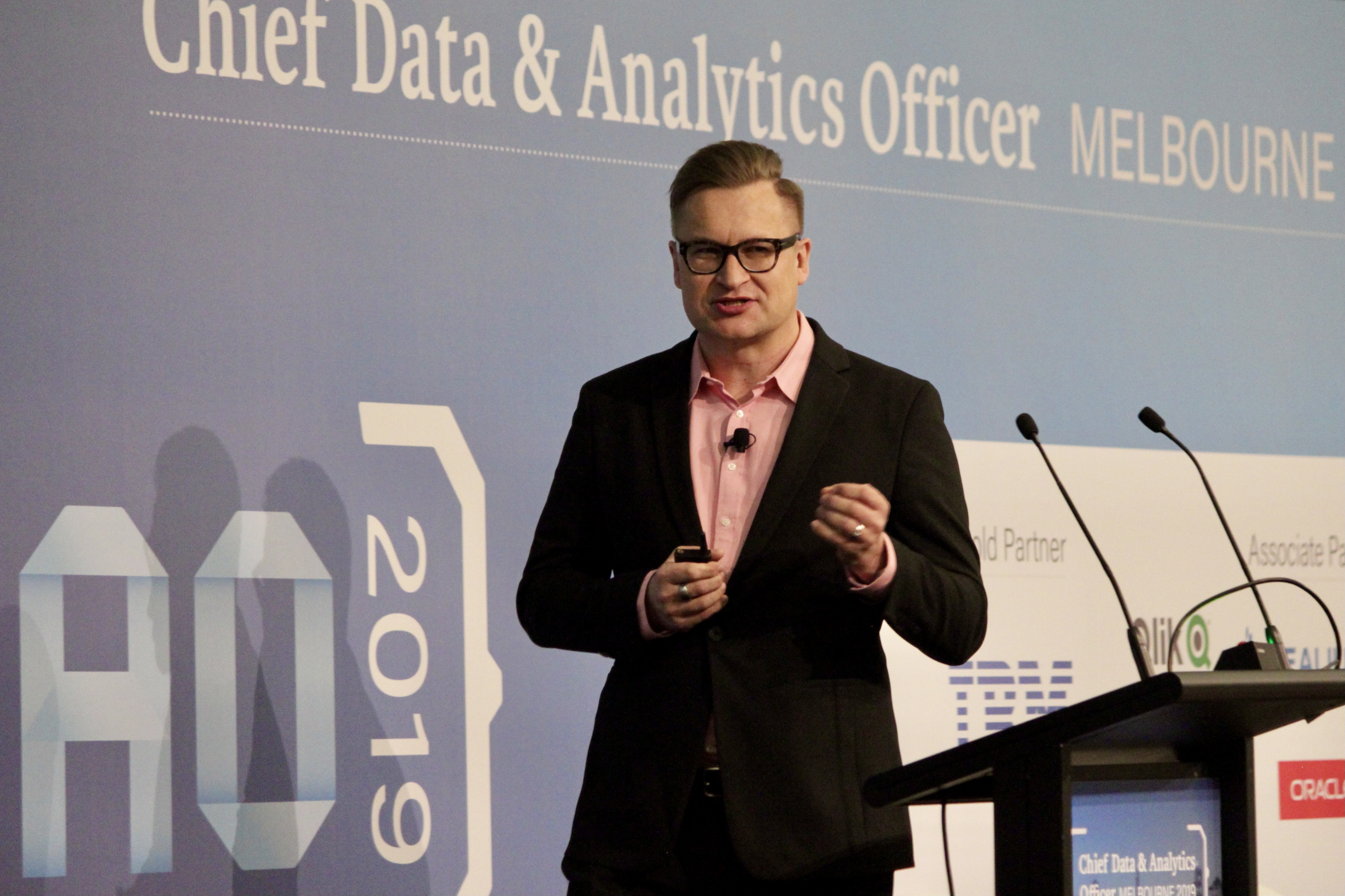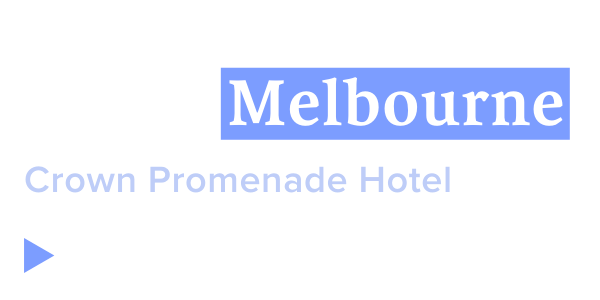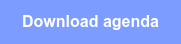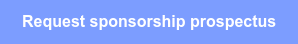## Be inspired, collaborate, disruptNow in its seventh year, we are thrilled to bring together Australia's largest gathering of Senior Level Data and Analytics Executives to explore what 2022 will have in store.

CDAO Melbourne 2022 will deliver THE industry roadmap for achieving data and analytics success.

We will focus on the biggest challenges faced today to effectively harness data as a strategic asset, and shape organisations to become analytically mature enterprises that outperform their competitors.

Key themes for this year include awakening data investment, managing data risk through strong governance, and sustaining and scaling data success.## Check out our latest CDAO Sydney event!50

## Expert speakers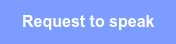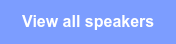## 2022 Key themes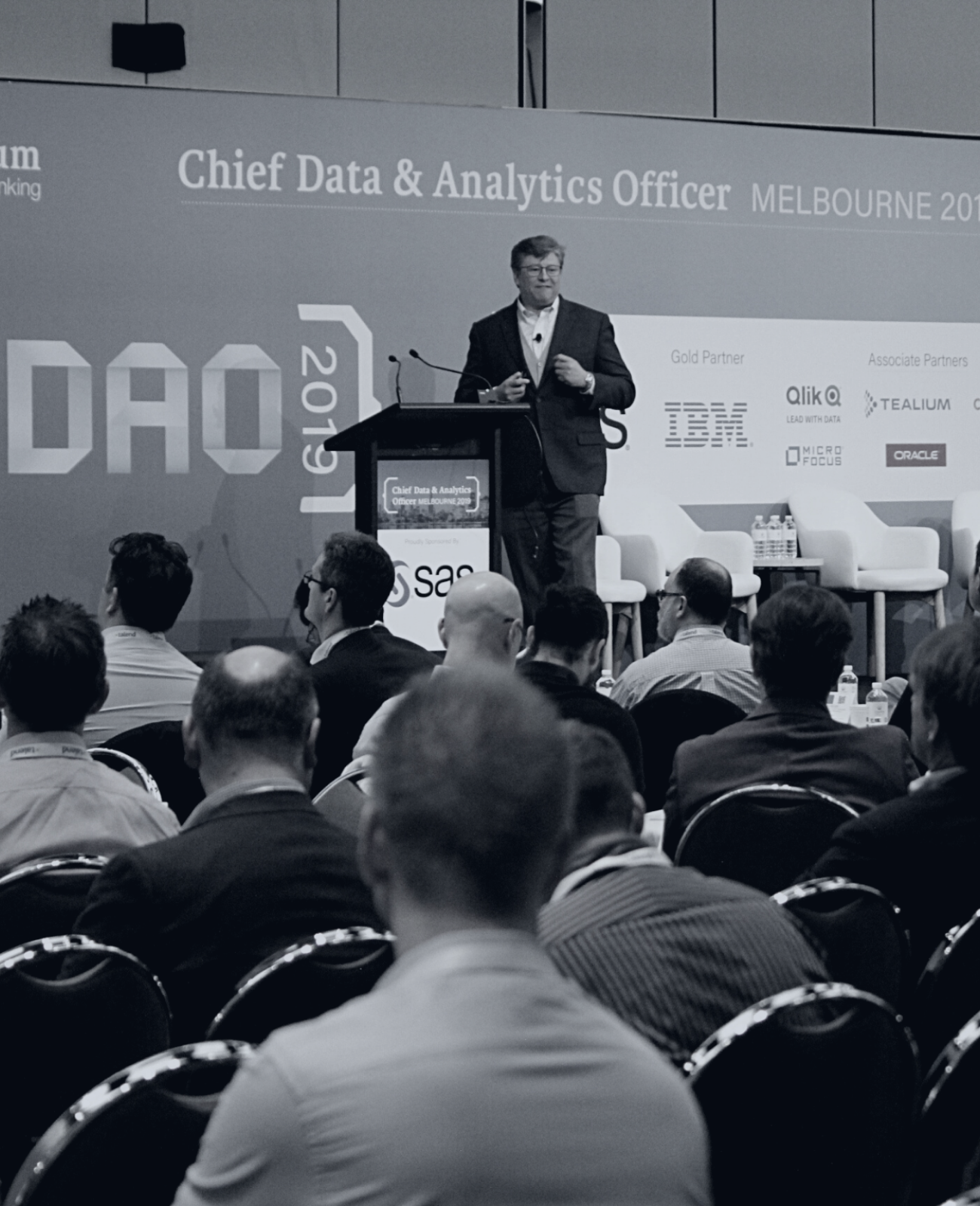Sustain change and scale for success
Ensure your strategy, foundational capabilities and activities are all geared towards embedding analytics into the fabric of the organisation.

Data-informed
Upskill, adapt and evolve. Learn how organisations are enabling change through entire cross-functional, data literate, agile teams.

Awaken data investment and opportunity, and treat data as a strategic asset
Turn your analytics narrative into a success story. With data analytics at a crossroads it’s time to separate the grain from the chaff and ensure value.

Thrive through change: Achieve data and analytics success in a post-COVID world
During the COVID-19 pandemic, data was the lifeblood of every country’s response. This event will look at how data was used, and how it will be deployed in the post-COVID world.

Effective risk management for data
Understand specific instruments and tools that can be deployed to operationalise data governance and ensure security, privacy and the ethical use of data and AI.## Experience CDAO Melbourne's onsite events

Connect and learn even faster at our onsite events. Every year we curate new experiences at the event to enhance relationships and spark ideas outside of the session room.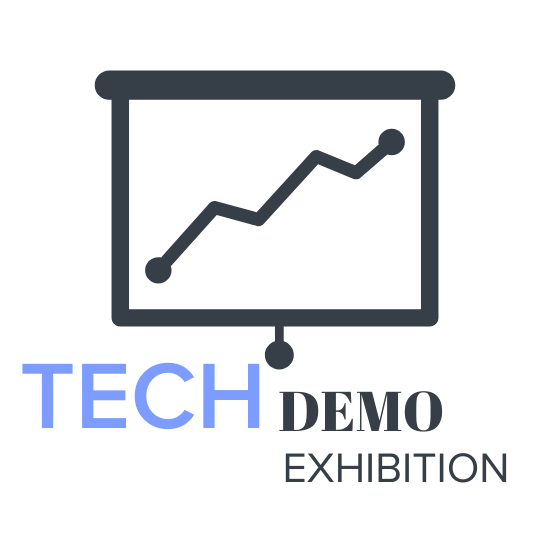Be inspired by those delivering change and serving the community through exciting new services.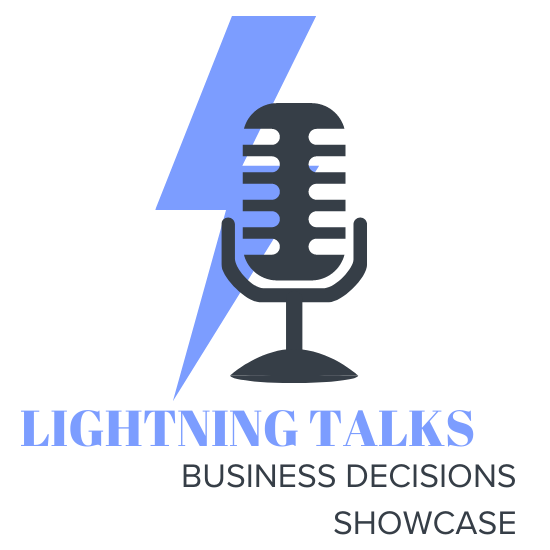Two organisations will take you through their project for 15 minutes, no waffle all substance.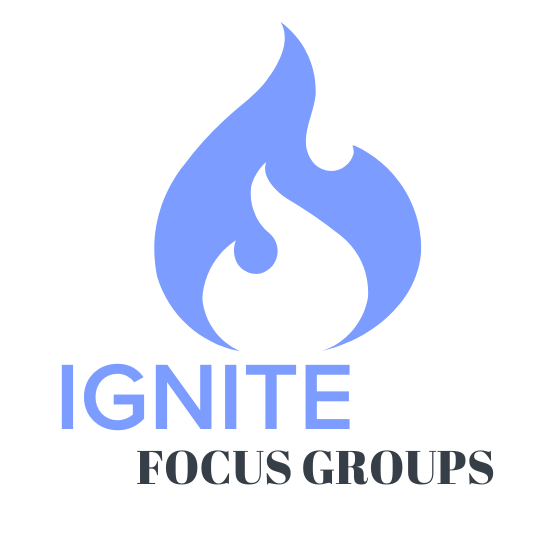Meet up with peers to discuss topics that most interest you!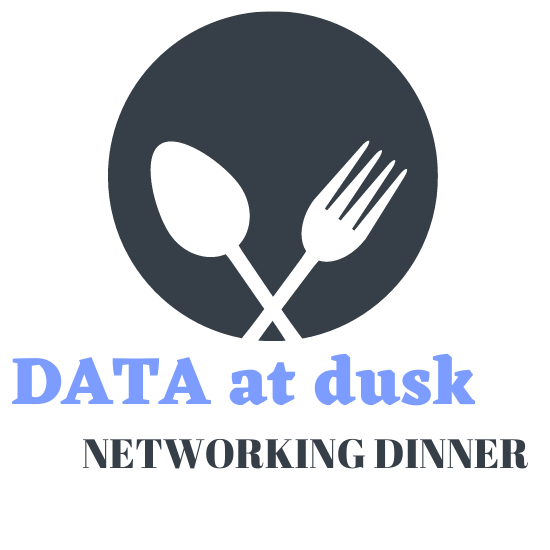Expand your C-level network at our exclusive, must-attend dinner.Bring discussions to life and have common problems solved in our no holds barred discussion groups.Relax, continue the conversation during an informal and fun cheers with peers.

## FEATURED REPORT: The Digital Opportunities in Healthcare in APAC 2022'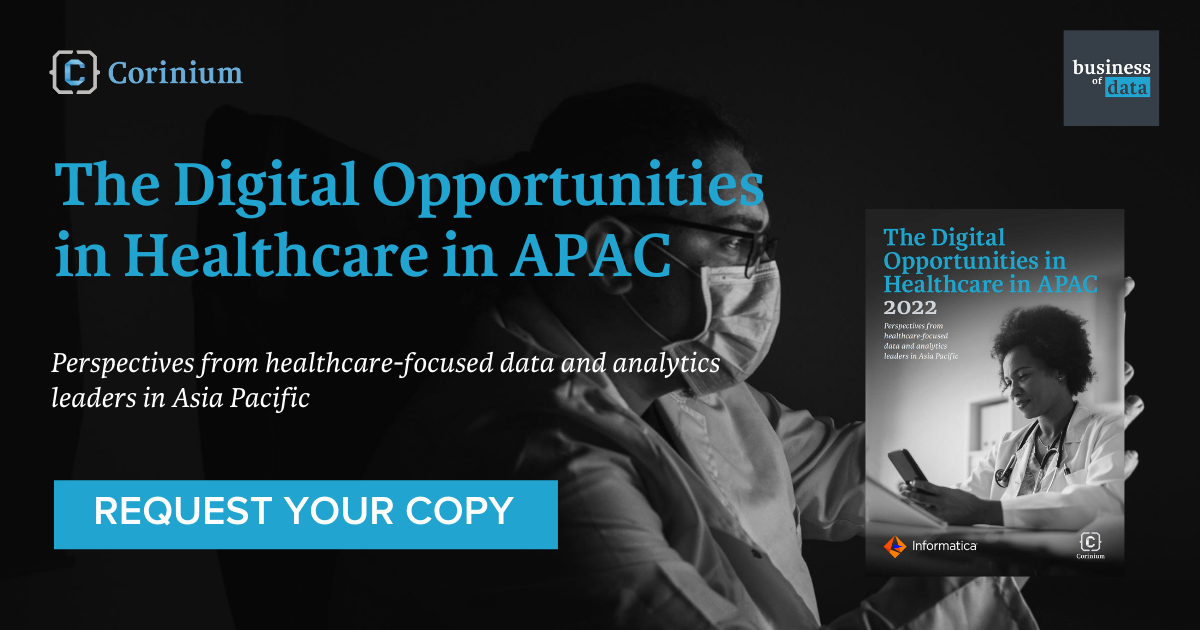Featuring insights from six health-focused data and analytics leaders across Asia-Pacific, this report explores the importance of data in healthcare, how it is put to work today, as well as what some exciting applications and projects coming together in the region are.

Get your hands on it by signing up here!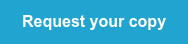## Thanks to our 2022 partners

true true true true true true true true true true true true true true true true true true true true true true true true true true true true true true true true true true true true true true true true true true true true true true true true true true true true true true true true true true true true true true true true true true true true true true true true true true true true true true true true true true true true true true true true true true true true true true true true true true true true true true true true true true true true true true true true true true true true true true true true true true true true true true true true true true true true true true true true true true true true true true true true true true true true true true true true true true true true true true true true true true true true true true true true true true true true true true true true true true true true true true true true true true true true true true true true true true true true true true true true true true true true true true true true true true true true true true true true true true true true true true true true true true true true true true true true true true true true true true true true true true true true true true true true true true true true true true true true true true true true true true true true true true true true true true true true true true true true true true true true true true true true true true true true true true true true true true true true true true true true true true true true true true true true true true true true true true true true true true true true true true true true true true true true true true true true true true true true true true true true true true true true true true true true true true true true true true true true true true true true true true true true true true true true true true true true true true true true true true true true true true true true true true true true true true true true true true true true true true true true true true true true true true true true true true true true true true true true true true true true true true true true true true true true true true true true true true true true true true true true true true true true true true true true true true true true true true true true true true true true true true

## What people are saying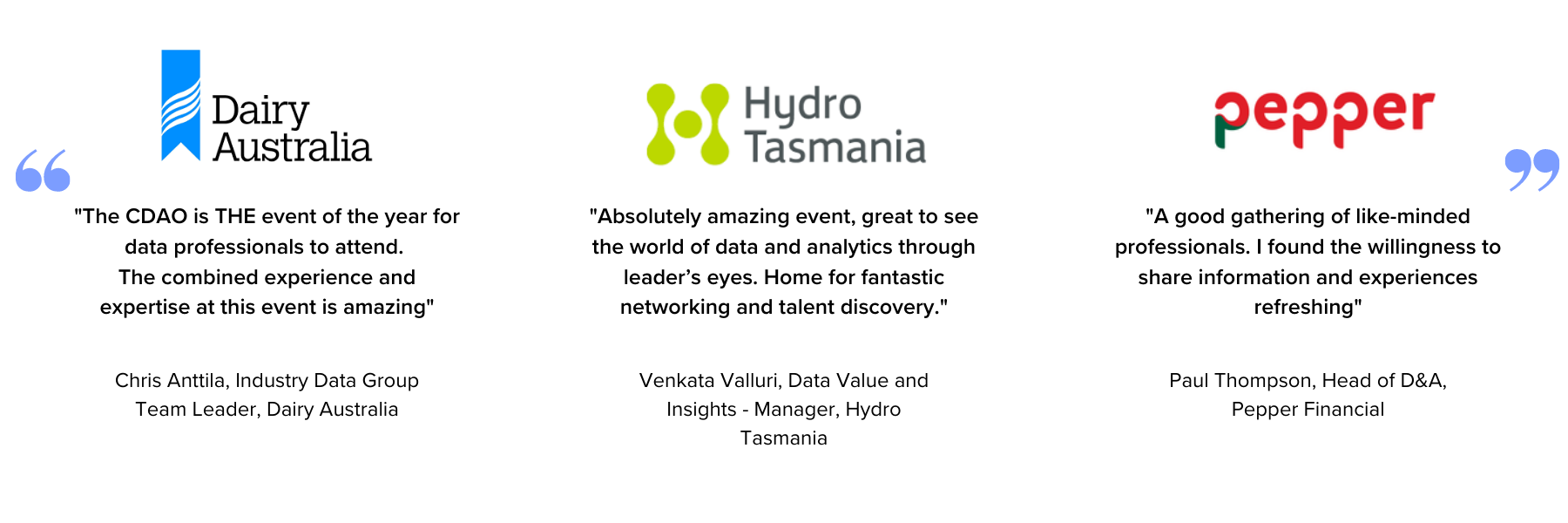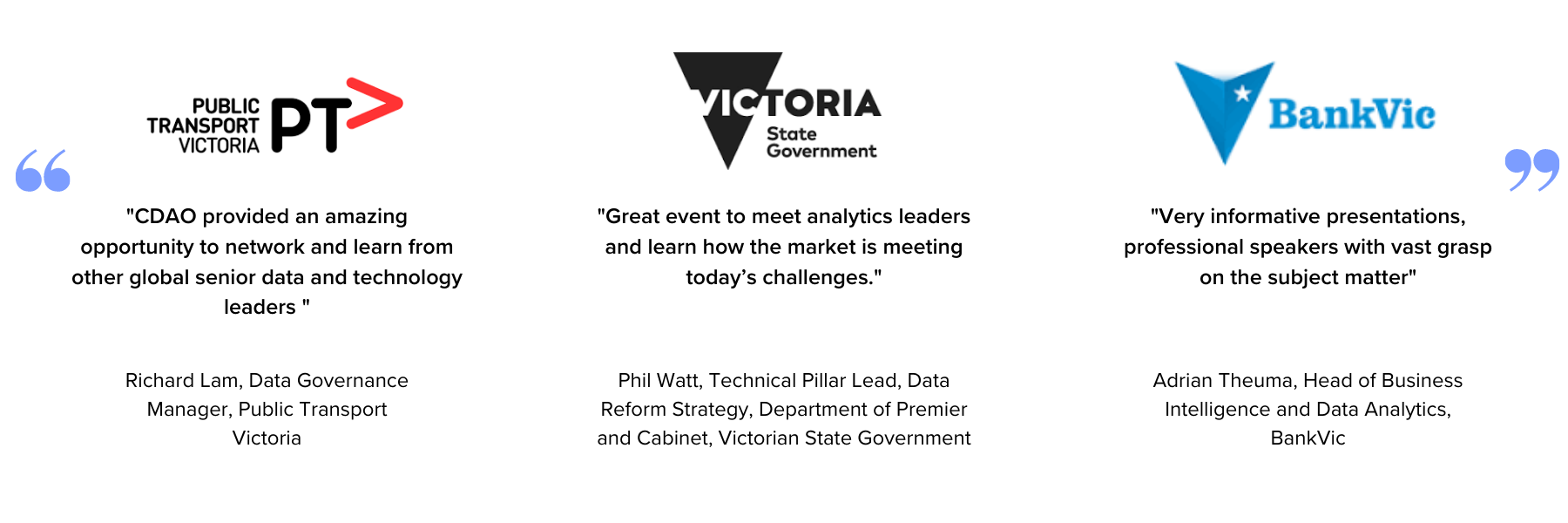## Previous events gallery